# Degree of Operating Leverage

The degree of operating leverage (DOL) is a measure of leverage used in corporate finance to analyze a company. Operating leverage magnifies the effect of changes in sales on operating earnings. The measure can be used to analyze a company’s sensitivity to changes in sales. DOL is often combined with two other measures of leverage, the degree of financial leverage (DFL) and the degree of total leverage (DTL).

On this page, we discuss the definition of DOL, discuss how to compute the degree of operating leverage, and finally provide an Excel spreadsheet which implements a degree of operating leverage calculator.

## Degree of operating leverage definition

What is the degree of operating leverage? DOL is defined as the percentage change in operating income (EBIT) that results from a given percentage change in revenues or sales. It may be easier to grasp this definition by using a formula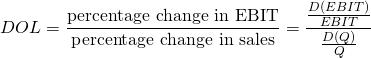The above formula may be still too abstract for some. Suppose we know the quantity of goods sold, then we can calculate DOL using the formula described below.

## Degree of operating leverage formula

How to compute the degree of operating leverage in practice? If we have Q, we can solve for the degree of DOL as follows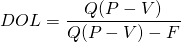Where Q is the quantity of goods sold, P is the price per unit, V is the variable cost per unit, and F is the fixed costs.

Rearranging, we get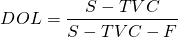where S is sales, TVC is total variable costs, and F is fixed costs.

Based on the above definition, firms with a high DOL are firms for which small changes in sales have a very big impact on whether the company’s net income is positive or negative.

## DOL example

To illustrate the above formulas, let’s consider an actual degree of operating leverage example.

Suppose we have a company who sells goods at a price of $4, has variable cost of$3 per unit, has fixed costs of $40,000 and generates$400,000 in sales. Then the DOL equals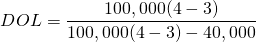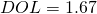This example illustrates that it is very easy to calculate DOL. We implement the above formulas using an Excel spreadsheet. The DOL calculator can be downloaded at the bottom of the page.

Finally, one word of caution. It is important to understand that the DOL is not constant. In particular, it changes with the number of units sold (Q). This is because the fixed costs F don’t change with production. Thus, the more a given company produces, the lower the DOL will be.

## Summary

We discussed DOL, an important measure used in accounting to measure the sensitivity of a company’s EBIT to changes in sales. A spreadsheet that implemetns the above formulas is available below.